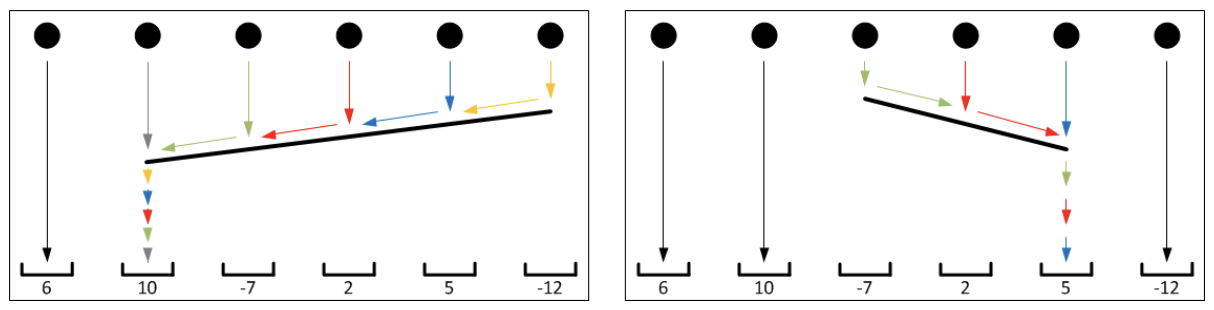시간 제한메모리 제한제출정답맞힌 사람정답 비율
1 초 128 MB396826623.239%

## 문제

There is one standard coordinate system with n balls and n holes in it: balls are located at positions (1, h), (2, h), …, (n, h), for some h > 0, and holes are located at (1, 0), (2, 0), …, (n, 0). At a given moment, all balls start falling down (along the y-axis, in the negative direction). For each ball that falls into the i-th hole, we get ci points. Of course, in order to avoid trivial c1 + c2 + … + cn score, it is allowed to put an obstacle.

A left obstacle is a segment (x1, y1) - (x2, y2) with properties: x1, x2 ∈ N, 1 ≤ x1 < x2 ≤ n and 0 < y1 < y2 < h (i.e. obstacle is tilted to the left). A right obstacle is a segment (x1, y1) - (x2, y2) with properties: x1, x2 ∈ N, 1 ≤ x1 < x2 ≤ n and h > y1 > y2 > 0 (i.e. obstacle is tilted to the right). If a ball, during its fall, hits an obstacle (segment), the ball temporarily stops, then slides to the obstacle’s lower end and, finally, falls directly into the hole under the obstacle’s lower end.

The left image shows trajectories of balls when the left obstacle is set, while on the right image we can see ball’s trajectories when a right obstacle is set. Notice that when an obstacle is set, there are holes with multiple balls in them and holes with no balls in them; however, every ball falls into one of the holes.• What is the highest possible score you can achieve if you must set exactly one right obstacle?
• What is the highest possible score you can achieve if you must set exactly one left obstacle?

## 입력

The first line of input contains one integer n - the number of balls and holes. The second line of input contains n integers ci delimited by spaces - the values of the holes, ordered from left to right.

## 출력

In the first line of output write a single integer – the answer to the first question. In the second line of output write a single integer – the answer to the second question. You should use a 64-bit integer data type.

## 제한

• 3 ≤ n ≤ 300 000.
• -109 ≤ ci ≤ 109.

## 예제 입력 1

6
6 10 -7 2 5 -12


## 예제 출력 1

19
56


## 힌트

The optimal setting of right / left obstacles is shown in the images. By setting the right obstacle as shown in the right image, balls 3, 4 and 5 will fall down into hole number 5 and the score will be 6 + 10 + 5 + 5 + 5 + (-12) = 19 points. Analogously, by setting left obstacle as on the left image, we get 6 + 10 + 10 + 10 + 10 + 10 = 56 points. It is not possible to score more points with any different obstacle setting.

## 출처

• 데이터를 추가한 사람: cgiosy